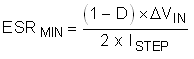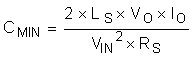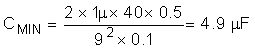SNVS480J January   2007  – July 2020

PRODUCTION DATA.

1. Features
2. Applications
3. Description
1.     Device Images
4. Revision History
5. Pin Configuration and Functions
6. Specifications
7. Detailed Description
1. 7.1 Overview
2. 7.2 Functional Block Diagram
3. 7.3 Feature Description
4. 7.4 Device Functional Modes
8. Application and Implementation
1. 8.1 Application Information
2. 8.2 Typical Application
1. 8.2.1 Design Requirements
2. 8.2.2 Detailed Design Procedure
3. 8.2.3 Application Curves
9. Power Supply Recommendations
10. 10Layout
1. 10.1 Layout Guidelines
2. 10.2 Layout Examples
11. 11Device and Documentation Support
12. 12Mechanical, Packaging, and Orderable Information

• DGS|10

#### 8.2.2.7 Input Capacitor

The input capacitors to a boost regulator control the input voltage ripple (ΔVIN) hold up the input voltage during load transients and prevent impedance mismatch (also called power supply interaction) between the LM5022 and the inductance of the input leads. Selection of input capacitors is based on their capacitance, ESR, and RMS current rating. The minimum value of ESR can be selected based on the maximum output current transient, ISTEP, using Equation 31.

Equation 31.For this example, the maximum load step is equal to the load current or 0.5 A. The maximum permissable ΔVIN during load transients is 4%P-P. ΔVIN and duty cycle are taken at minimum input voltage to give the worst-case value in Equation 32.

Equation 32. ESRMIN = [(1 – 0.77) × 0.36] / (2 × 0.5) = 83 mΩ

The minimum input capacitance can be selected based on ΔVIN, based on the drop in VIN during a load transient, or based on prevention of power supply interaction. In general, the requirement for greatest capacitance comes from the power supply interaction. The inductance and resistance of the input source must be estimated, and if this information is not available, they can be assumed to be 1 µH and 0.1 Ω, respectively. Minimum capacitance is then estimated with Equation 33.

Equation 33.As with ESR, the worst-case, highest minimum capacitance calculation comes at the minimum input voltage. Using the default estimates for LS and RS, minimum capacitance is calculated with Equation 34.

Equation 34.The next highest standard 20% capacitor value is 6.8 µF, but because the actual input source impedance and resistance are not known, two 4.7-µF capacitors is used. In general, doubling the calculated value of input capacitance provides a good safety margin. The final calculation is for the RMS current. For boost converters operating in CCM, this can be estimated with Equation 35.

Equation 35. IRMS = 0.29 × ΔiL(MAX)

From the inductor section, maximum inductor ripple current is 0.58 A, hence the input capacitor or capacitors must be rated to handle 0.29 × 0.58 = 170 mARMS.

The input capacitors can be ceramic, tantalum, aluminum, or almost any type, however, the low capacitance requirement makes ceramic capacitors particularly attractive. As with the output capacitors, the minimum quality dielectric used must be X5R, with X7R or better preferred. The voltage rating for input capacitors requirement does not need to be as conservative as the output capacitors, as the requirement for capacitance decreases as input voltage increases. For this example, the capacitor selected is 4.7 µF ±20%, rated to 50 V in the 1812 case size. The RMS current rating of these capacitors is over 2 A each, more than enough for this application.